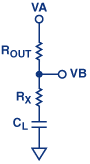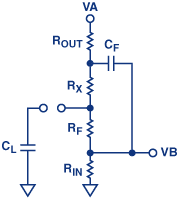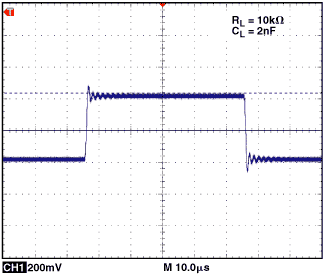# Ask The Application Engineer—32: Practical Techniques to Avoid Instability Due to Capacitive Loading

Q: ADI has published a lot of information on dealing with capacitive loading and other stability issues in books, such as the amplifier seminar series, in earlier issues of Analog Dialogue, and in some design tools. But, I need a refresher—NOW.

A: OK. Here goes!

Capacitive loads often give rise to problems, in part because they can reduce the output bandwidth and slew rate, but mainly because the phase lag they produce in the op amp’s feedback loop can cause instability. Although some capacitive loading is inevitable, amplifiers are often subjected to sufficient capacitive loading to cause overshoots, ringing, and even oscillation. The problem is especially severe when large capacitive loads, such as LCD panels or poorly terminated coaxial cables, must be driven—but unpleasant surprises in precision low-frequency and dc applications can result as well.

As will be seen, the op amp is most prone to instability when it is configured as a unity-gain follower, either because (a) there is no attenuation in the loop, or (b) large common-mode swings, though not substantially affecting accuracy of the signal gain, can modulate the loop gain into unstable regions.

The ability of an op amp to drive capacitive loads is affected by several factors:

1. the amplifier’s internal architecture (for example, output impedance, gain and phase margin, internal compensation circuitry)
2. the nature of the load impedance
3. attenuation and phase shift of the feedback circuit, including the effects of output loads, input impedances, and stray capacitances.

Among the parameters cited above, the amplifier output impedance, represented by the output resistance, RO, is the one factor that most affects performance with capacitive loads. Ideally, an otherwise stable op amp with RO = 0 will drive any capacitive load without phase degradation.

To avoid sacrificing performance with light loads, most amplifiers are not heavily compensated internally for substantial capacitive loads, so external compensation techniques must be used to optimize those applications in which a large capacitive load at the output of the op amp must be handled. Typical applications include sample-and-hold amplifiers, peak detectors, and driving unterminated coaxial cables.

Capacitive loading, as shown in Figures 1 and 2, affects the open-loop gain in the same way, regardless of whether the active input is at the noninverting or the inverting terminal: the load capacitance, CL, forms a pole with the open-loop output resistance, RO. The loaded gain can be expressed as follows:

and A is the unloaded open-loop gain of the amplifier.

The –20 dB/decade slope and 90° lag contributed by the pole, added to the –20 dB slope and 90° contributed by the amplifier (plus any other existing lags), results in an increase in the rate of closure (ROC) to a value of at least 40 dB per decade, which, in turn, causes instability.

This note discusses typical questions about the effects of capacitive loads on the performance of some amplifier circuits, and suggests techniques to solve the instability problems they raise.Figure 1. A simple op amp circuit with capacitive load.Figure 2. Bodé plot for the circuit of Figure 1.

Q: So, different circuits call for different techniques?

A: Yes, absolutely! You’ll choose the compensation technique that best suits your design. Some examples are detailed below. For example, here’s a compensation technique that has the added benefit of filtering the op amp’s noise via an RC feedback circuit.Figure 3. In-the-loop compensation circuit.

Figure 3 shows a commonly used compensation technique, often dubbed in-the-loop compensation. A small series resistor, Rx, is used to decouple the amplifier output from CL; and a small capacitor, Cf, inserted in the feedback loop, provides a high frequency bypass around CL.

To better understand this technique, consider the redrawn feedback portion of the circuit shown in Figure 4. VB is connected to the amplifier’s minus input.Figure 4. Feedback portion of the circuit.

Think of the capacitors, Cf  and CL, as open circuits at dc, and shorts at high frequencies. With this in mind, and referring to the circuit in Figure 4, let’s apply this principle to one capacitor at a time.

Case 1 (Figure 5a):

With Cf shorted, Rx << Rf, and Ro << Rin, the pole and zero are functions of CL, Ro, and Rx.Figure 5a. Cf short-circuited.

Thus,

and

Case 2. (Figure 5b):

With CL open, the pole and zero are a function of Cf.Figure 5b. CL open-circuited.

Thus,

By equating the pole in Case 1 to the zero in Case 2, and the pole in Case 2 to the zero in Case 1, we derive the following two equations:

The formula for Cf includes the term, Acl (amplifier closed-loop gain, 1+Rf/Rin). By experimenting, it was found that the 1/Acl term needed to be included in the formula for Cf. For the above circuit, these two equations alone will allow compensation for any op amp with any applied capacitive load.

Although this method helps prevents oscillation when heavy capacitive loads are used, it reduces the closed-loop circuit bandwidth drastically. The bandwidth is no longer determined by the op amp, but rather by the external components, Cf and Rf, producing a closed-loop bandwidth of: f–3 dB = 1/(2πCfRf).

A good, practical example of this compensation technique can be seen with the AD8510, an amplifier that can safely drive up to 200 pF while still preserving a 45° phase margin at unity-gain crossover. With the AD8510 in the circuit of Figure 3, configured for a gain of 10, with a 1-nF load capacitance at the output and a typical output impedance of 15 ohms, the values of Rx and Cf, computed using the above formulas, are 2 ohms and 2 pF. The square wave responses of Figures 6 and 7 show the fast response with uncompensated ringing, and the slower, but monotonic corrected response.Figure 6. AD8510 output response without compensation.Figure 7. AD8510 output response with compensation.

In Figure 7, note that, because Rx is inside the feedback loop, its presence does not degrade the dc accuracy. However, Rx should always be kept suitably small to avoid excessive output swing reduction and slew-rate degradation.

Caution: The behaviors discussed here are typically experienced with the commonly used voltage-feedback amplifiers. Amplifiers that use current feedback require different treatment—beyond the scope of this discussion. If these techniques are used with a current feedback amplifier, the integration inherent in Cf will cause instability.

### Out-of-the-Loop Compensation

Q: Is there a simpler compensation scheme that uses fewer components?

A: Yes, the easiest way is to use a single external resistor in series with the output. This method is effective but costly in terms of performance (Figure 8).Figure 8. External Rseries isolates the amplifier’s feedback loop from the capacitive load.

Here a resistor, Rseries, is placed between the output and the load. The primary function of this resistor is to isolate the op-amp output and feedback network from the capacitive load. Functionally, it introduces a zero in the transfer function of the feedback network, which reduces the loop phase shift at higher frequencies. To ensure a good level of stability, the value of Rseries should be such that the zero added is at least a decade below the unity-gain crossover bandwidth of the amplifier circuit. The required amount of series resistance depends primarily on the output impedance of the amplifier used; values ranging from 5 ohms to 50 ohms are usually sufficient to prevent instability. Figure 9 shows the output response of the OP1177 with a 2-nF load and a 200-mV peak-peak signal at its positive input. Figure 10 shows the output response under the same conditions, but with a 50-ohm resistor in the signal path.Figure 9. Output response of follower-connected OP1177 with capacitive load. Note high frequency ringing.Figure 10. OP1177 output response with 50-ohm series resistance. Note reduced ringing.

The output signal will be attenuated by the ratio of the series resistance to the total resistance. This will require a wider amplifier output swing to attain full-scale load voltage. Nonlinear or variable loads will affect the shape and amplitude of the output signal.

### Snubber Network

Q: If I’m using a rail-to-rail amplifier, can you suggest a stabilizing method that will preserve my output swing and maintain gain accuracy?

A: Yes, with an R-C series circuit from output to ground, the snubber method is recommended for lower voltage applications, where the full output swing is needed (Figure 11).Figure 11. The RS-CS load forms a snubber circuit to reduce the phase shift caused by CL.

Depending on the capacitive load, application engineers usually adopt empirical methods to determine the correct values for Rs and Cs. The principle here is to resistively load down the output of the amplifier for frequencies in the vicinity at which peaking occurs—thus snubbing down the amplifier’s gain, then use series capacitance to decrease the loading at lower frequencies. So, the procedure is to: check the amplifier’s frequency response to determine the peaking frequency; then, experimentally apply values of resistive loading (Rs) to reduce peaking to a satisfactory value; then, compute the value of Cs for a break frequency at about 1/3 the peak frequency. Thus, Cs = 3/(2πfpRs), where fp is the frequency at which peaking occurs.

These values can also be determined by trial and error while looking at the transient response (with capacitive loading) on an oscilloscope. The ideal values for Rs and Cs will yield minimum overshoot and undershoot. Figure 12 shows the output response of the AD8698 with a 68-nF load in response to a 400-mV signal at its positive input. The overshoot here is less than 25% without any external compensation. A simple snubber network reduces the overshoot to less than 10%, as seen in Figure 13. In this case, Rs and Cs are 30 ohms and 5 nF, respectively.Figure 12. AD8698 output response without compensation.Figure 13. AD8698 output response with snubber network.

Q: OK. I understand these examples about dealing with capacitive loading on the amplifier output. Now, is capacitance at the input terminals also of concern?

A: Yes, capacitive loading at the inputs of an op amp can cause stability problems. We’ll go through a few examples.

A very common and typical application is in current-to-voltage conversion when the op amp is used as a buffer/amplifier for a current-output DAC. The total capacitance at the input consists of the DAC output capacitance, the op amp input capacitance, and the stray wiring capacitance.

Another popular application in which significant capacitance may appear at the inputs of the op amp is in filter design. Some engineers may put a large capacitor across the inputs (often in series with a resistor) to prevent RF noise from propagating through the amplifier—overlooking the fact that this method can lead to severe ringing or even oscillation.

To better understand what is going on in a representative case, we analyze the circuit of Figure 14, unfolding the equivalent of its feedback circuit (input, Vin, grounded) to derive the feedback transfer function:

which gives a pole located atFigure 14. Capacitive loading at the input—inverting configuration.

This function indicates that the noise gain (1/β) curve rises at 20 dB/decade above the break frequency, fp. If fp is well below the open-loop unity-gain frequency, the system becomes unstable. This corresponds to a rate of closure of about 40 dB/decade. The rate of closure is defined as the magnitude of the difference between slopes of the open-loop gain (dB) plot (–20 dB/decade at most frequencies of interest) and that of 1/β, in the neighborhood of the frequency at which they cross (loop gain = 0 dB).

To cure the instability induced by C1, a capacitor, Cf, can be connected in parallel with R2, providing a zero which can be matched with the pole, fp, to lower the rate of closure, and thus increase the phase margin. For a phase margin of 90°, pick Cf =(R1/R2)C1.

Figure 15 shows the frequency response of the AD8605 in the configuration of Figure 14.Figure 15. Frequency response of Figure 14.

Q: Can I predict what the phase margin would be, or how much peaking I should expect?

A: Yes, here’s how:

You can determine the amount of uncompensated peaking using the following equation:

where fu is the unity gain bandwidth, fz is the breakpoint of the 1/β curve, and C1 is the total capacitance—internal and external—including any parasitic capacitance.

The phase margin (Φm) can be determined with the following equation:

The AD8605 has a total input capacitance of approximately 7 pF. Assuming the parasitic capacitance is about 5 pF, the closed-loop gain will have a severe peaking of 5.5 dB, using the above equation. In the same manner, the phase margin is about 29°, a severe degradation from the op amp’s natural phase response of 64°.

Q: How can I make sure the op amp circuit is stable if I want to use an RC filter directly at the input?

A: You can use a similar technique to that described above. Here’s an example:

It is often desirable to use capacitance to ground from an amplifier’s active input terminals to reduce high-frequency interference, RFI and EMI. This filter capacitor has a similar effect on op amp dynamics as increased stray capacitance. Since not all op amps behave in the same way, some will tolerate less capacitance at the input than others. So, it is useful in any event, to introduce a feedback capacitor, Cf, as compensation. For further RFI reduction, a small series resistor at the amplifier terminal will combine with the amplifier’s input capacitance for filtering at radio frequencies. Figure 16 shows an approach (at left), that will have difficulty maintaining stability, compared with a considerably improved circuit (at right). Figure 17 shows their superimposed square wave responses.Figure 16. Input filter without (at left), and with (at right) compensation and lower impedance levels.Figure 17. Comparison of output responses of the circuits in Figure 16. The circuit at left resulted in the oscillatory response.

Q: You mentioned earlier that stray capacitance is added to the total input capacitance. How important is stray capacitance?

A: Unsuspected stray capacitance can have a detrimental impact on the stability of the op amp. It is very important to anticipate and minimize it.

The board layout can be a major source of stray input capacitance. This capacitance occurs at the input traces to the summing junction of the op amp. For example, one square centimeter of a PC board, with a ground plane surrounding it, will produce about 2.8 pF of capacitance (depending on the thickness of the board).

To reduce this capacitance: Always keep the input traces as short as possible. Place the feedback resistor and the input source as close as possible to the op amp input. Keep the ground plane away from the op amp, especially the inputs, except where it is needed for the circuit and the noninverting pin is grounded. When ground is really needed, use a wide trace to ensure a low resistance path to ground.

Q: Can op amps that aren’t unity-gain stable be used at unity-gain? The OP37 is a great amplifier, but it must be used in a gain of at least 5 to be stable.

A: You can use such op amps for lower gains by tricking them. Figure 18 shows a useful approach.Figure 18. Unity-gain follower using an input series R-C to stabilize an amplifier that is not stable at unity-gain.

In Figure 18, RB and RA provide enough closed-loop gain at high frequencies to stabilize the amplifier, and C1 brings it back to unity at low frequencies and dc. Calculating the values of RB and RA is fairly straightforward, based on the amplifier’s minimum stable gain. In the case of the OP37, the amplifier needs a closed-loop gain of at least 5 to be stable, so RB = 4RA for β = 1/5. For high frequencies, where C1 behaves like a direct connection, the op amp thinks it’s operating at a closed-loop gain of 5, and is therefore stable. At dc and low frequencies, where C1 behaves like an open circuit, there is no attenuation of negative feedback, and the circuit behaves like a unity-gain follower.

The next step is to calculate the value of capacitance, C1. A good value for C1 should be picked such that it will provide a break frequency at least a decade below the circuit’s corner frequency (f–3 dB).

Figure 19 shows the output of the OP37 in response to a 2-V p-p input step. The values of the compensation components are chosen using the equations above, with fc = 16 MHzFigure 19. Unity-gain response of the OP37 with and without compensation.

Q: Can this approach also be used for the inverting configuration? Can I still use the same equations?

A: For the inverting configuration, the analysis is similar, but the equations for the closed-loop gain are slightly different. Remember that the input resistor to the inverting terminal of the op amp is now in parallel with RA at high frequencies. This parallel combination is used to calculate the value of RA for minimum stable gain. The capacitance value, C1, is calculated in the same way as for the noninverting case.

Q: Are there drawbacks to using this technique?

A: Indeed, there are. Increasing the noise gain will increase the output noise level at higher frequencies, which may not be tolerable in some applications. Care should be used in wiring, especially with high source impedance, in the follower configuration. The reason is that positive feedback via capacitance to the amplifier’s noninverting input at frequencies where the gain is greater than unity, can invite instability, as well as increased noise.